Two-Zero Next  |  Prev  |  Up  |  Top  |  Index  |  JOS Index  |  JOS Pubs  |  JOS Home  |  Search

### Two-Zero

The signal flow graph for the general two-zero filter is given in Fig.10.8, and the derivation of frequency response is as follows: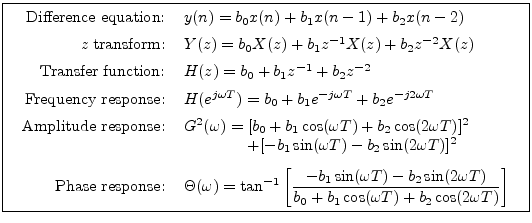The parameters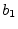and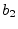are called the numerator coefficients, and they determine the two zeros. Using the quadratic formula for finding the roots of a second-order polynomial, we find that the zeros are located at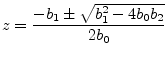If the zeros are real [], then the two-zero case reduces to two instances of our earlier analysis for the one-zero. Assuming the zeros to be complex, we may express the zeros in polar form as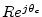and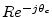, where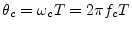.

Forming a general two-zero transfer function in factored form gives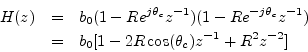from which we identify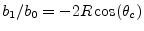and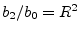, so that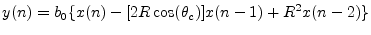is again the difference equation of the general two-zero filter with complex zeros. The frequency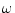, is now viewed as a notch frequency, or antiresonance frequency. The closer R is to 1, the narrower the notch centered at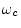.

The approximate relation between bandwidth and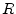given in Eq. (10.5) for the two-pole resonator now applies to the notch width in the two-zero filter.

Figure 10.9 gives some two-zero frequency responses obtained by setting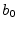to 1 and varying. The value of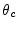, is again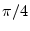. Note that the response is exactly analogous to the two-pole resonator with notches replacing the resonant peaks. Since the plots are on a linear magnitude scale, the two-zero amplitude response appears as the reciprocal of a two-pole response. On a dB scale, the two-zero response is an upside-down two-pole response.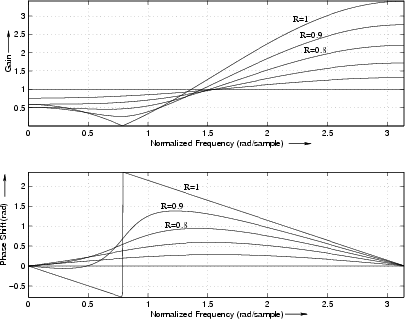Next  |  Prev  |  Up  |  Top  |  Index  |  JOS Index  |  JOS Pubs  |  JOS Home  |  Search

[How to cite this work] [Order a printed hardcopy]